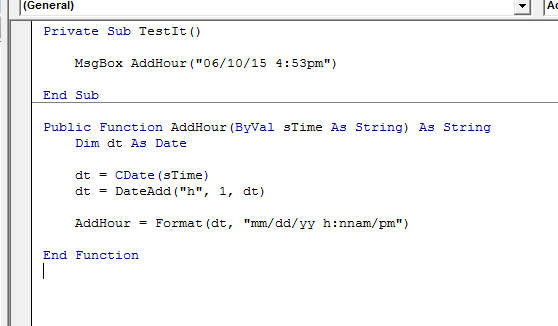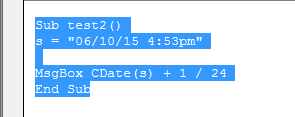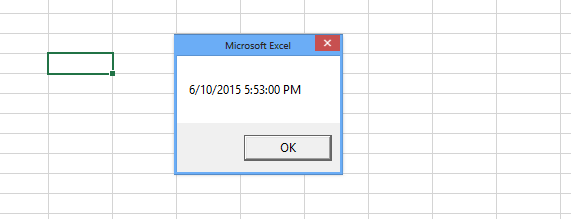#### How to Add Hours to Time in Excel Vba

In Excel VBA, you can add hours to a date and time value using the DateAdd function. The DateAdd function allows you to perform various time arithmetic operations, including adding hours.

## Basic declarations

Let’s start with the basic declarations in Excel. We can declare the date variable as follows:

`Dim date1 as Date`

Now we can pass a date to this variable as

`date1 = "11/03/2020"`

But we can also pass the time along with a date, which will be saved in the variable type:

`date1 = "11/03/2020 4:53pm"`

Now we want to add hours to this variable, which has got the date and time together.

Suppose we want to add 1 hour to date1. We can not directly add it like this:

` Date1 = date1 + 1`

which is not correct.

So we are using the following function and sub for the same:

```Private Sub TestIt()
Public Function AddHour(ByVal sTime As String) As String
Dim dt As Date

dt = CDate(sTime)

End Function```In this function, we are declaring the variable dt as the date and then the value “06/10/15 4:53pm” is passed to this variable from the sub.

This function uses an inbuilt function called Dateadd to add 1 hour, and then the result is formatted as the input format.

The x function is used to perform time arithmetic in Excel VBA. With the Dateadd function, you can add not only hours, but also year, quarter, month, day of the year, day, day of the week, week, minute, second.

The syntax of Dateadd function is:

`DateAdd (interval, number, date)`

The format of the interval in the dateadd function is:

• yyyy Year
• q Quarter
• m Month
• y Day of the year
• d Day
• w Weekday
• ww Week
• h Hour
• n Minute
• s Second

In the above case, I was adding hours to time, so I used the interval h.

## Simple way

We can also use another simple way. Add a simple msgbox and program it using this vba code.

```Sub test2()
s = "06/10/15 4:53pm"
MsgBox CDate(s) + 1 / 24
End Sub```It will also give the same result.To make the code more readable and maintainable, use variables to store date and time values, especially if you need to perform multiple calculations.

```Dim myDateTime As Date
myDateTime = CDate("06/10/15 4:53pm")
myDateTime = myDateTime + 1 / 24
MsgBox myDateTime
```

Ensure that the input date and time string is in a format that VBA can recognize. The format “mm/dd/yy hh:nnam/pm” is a common choice, but you may need to adjust it based on your specific input format.

By following these tips and understanding how to use the DateAdd function, you can effectively perform date and time calculations in Excel VBA, including adding hours to date-time values.